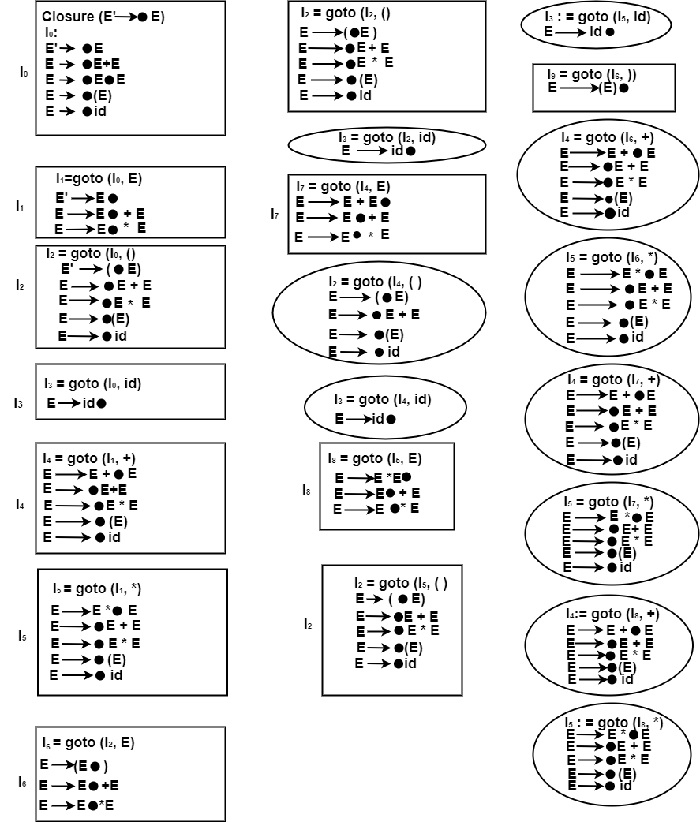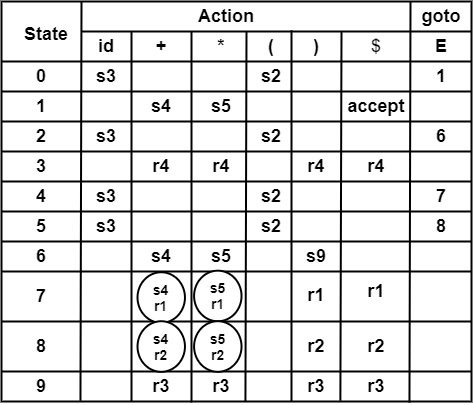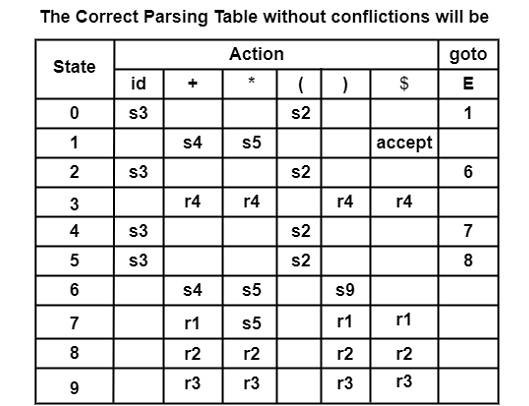# Consider the ambiguous grammar. E → E + E E → E * E E → (E) E → id (a) Construct LR (0) items for above grammar. (b) Construct SLR parsing table for grammar. (c) Parse the input string id + id * id.

Compiler DesignProgramming LanguagesComputer Programming

#### Web Design for Beginners: Build Websites in HTML & CSS 2022

68 Lectures 8 hours

#### HTML5 & CSS3 Site Design

61 Lectures 6 hours

## Solution

Step1− Construct Augmented Grammar

(0) E′ → S

(1) E → E + E

(2) E → E ∗ E

(3) E → (E)

(4) E → id

Step2− Find closure & goto functions to construct LR (0) items.

Closure (E′ → ∙ E) =Applying goto on I9

∵ goto cannot be applied on I9, as the dot in E → (E). is on the last position.

Step3− Computation of FOLLOW

Applying Rule (1) FOLLOW

FOLLOW(B) = {$} (1) • E → E + E Comparing E → E + E with A → α B β ∴ α = ε, B = E, β = +E ∵ FIRST(β) = FIRST(+E) = {+} ∴ Rule (2a)of FOLLOW FOLLOW(E) = {+} (2) Applying Rule (3) Comparing E → E + E with A → α B ∴ A = E, α = E+, B = E FOLLOW(E) = {FOLLOW(E)} (3) • E → E ∗ E Applying Rule (2) and Rule (3) of FOLLOW, we get FOLLOW(E) = {*} (4) FOLLOW(E) = {FOLLOW(E)} (5) • E → (E) Applying Rule (2) Comparing E → (E) with A → α B β ∴ FOLLOW (E) = {)} (6) Rule (3) cannot be applied to this production As E → (E) cannot be compared with A → α B • E → id Rule (2) and (3) of FOLLOW cannot be applied on E → id. As E → id cannot be compared with A → α B β and A → α B. Combining (1) to (6), we get FOLLOW (E) = {$, +,*, )}

Step4 − Construction of SLR Parsing TableIn the parsing table, conflict occurs at Row state 7, 8, and column *, +.

In Action [7, +],                          Action [7, *]

Action [8, +],                             Action [8, *] there occurs a shift-reduce conflict.

The Association and precedence rules can remove this conflict.

Parsing the string id + id * id

StackInputAction
0id + id * id $Shift 0 id 3+ id * id$Reduce by E → id
0 E 1+id * id $Shift 0 E 1 + 4id * id$Shift
0 E 1 + 4 id 3* id $Reduce by E → id 0 E 1 + 4 E 7* id$Conflict i. e. , s5 or r1 ∴ * has higher precedence then + ∴ Action [7,∗] = s5 So, shift-reduce, i.e., s5
0 E 1 + 4 E 7 * 5$Shift 0 E 1 + 4 E 7 * 5 id 3$Reduce by E → id
0 E 1 + 4 E 7 * 5 E 8$Reduce by E → E * E 0 E 1 + 4 E 7$Reduce by E → E + E
0 E 1$accept The above parsing solves the conflicting problem in Action [7, *]. So, Action [7, *] = s5 instead of r1. Similarly on Parsing the string id + id + id. StackInputAction 0id + id + id$Shift
0 id 3+ id + id $Reduce by E → id 0 E 1+id + id$Shift
0 E 1 + 4id + id $Shift 0 E 1 + 4 id 3+id$Reduce by E → id
0 E 1 + 4 E 7+id $Conflict i. e. , Action [7, +] = s4 or r1. ∴ + is Associative (left). 0E1 + 4E7 will be reduced before shifting + ∴ Action [7, +] = r1 ∴ Reduce by E → E + E 0 E 1+id$Shift
0 E + 4id $Shift 0 E + 4 id 3$Reduce by E → id
0 E + 4 E 7$Reduce by E → E + E 0 E 1$accept

So, the Above parsing shows how to resolve shift Reduce conflict at Action [7, +]

So, Action [7, +] = r1 instead of s4

Similarly, other entries such as Action [8, +] and Action [8, *] can be solved by taking strings.

id * id * id and id * id + id

Resolution is                 Action [8, +] = r2 and

Action [8, *] = r2.